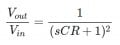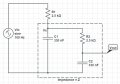# How can I drive the transfer function of a second order passive RC filter?

#### Michael George

Joined Feb 8, 2015
53
I'm good at math and complex numbers and I understand the transfer function of the first order passive low pass filter.
When I add two stages of first order passive low pass filter to form a second order filter, The loading effect appears because the two stages have mutual relationship.
I would like to know How to drive the transfer function of the second order low pass filter taking in consideration the loading effect.
I think the following equation is for Ideal filters only:Is there a link to website or a pdf that tells me how to get the transfer function of practical (real) filters, Please?

Thank you very much,

#### mcasale

Joined Jul 18, 2011
210
Did you try to Google something like "second order filter"? There should be tons of information.

•Michael George

#### Michael George

Joined Feb 8, 2015
53
Did you try to Google something like "second order filter"? There should be tons of information.
I give it a try and I found active filters, LC filters, calculators but I still can not find exactly what I need.

#### WBahn

Joined Mar 31, 2012
26,937
I don't know what it means to "drive" a transfer function. I assuming it was just a typo when you used it in the title, but then you used it again in the post. Did you actually mean "derive"? I'll assume that's the case unless you say differently.

The most convincing way to get the transfer function is to construct it directly from an analysis of the circuit. Have you given that a try? If not, do so. If you run into problems you can post your schematic and your analysis attempt and we will help you through it. You'll find it's not as hard as you are probably thinking.

•Michael George

#### Michael George

Joined Feb 8, 2015
53
@Jony130 The link is very useful. Thank you very much.

#### Michael George

Joined Feb 8, 2015
53
@WBahn I'm sorry about my English. I've done the following analysis before. Sometimes these analysis work, Other times it gives wrong results. I don't know if the wrong results are due to some mistakes in mathematical steps (such as: forgetting a negative sign) or They are due to wrong electrical Idea (I'm not using electrical principals in a correct way). So, Would you check it for me, Please? I know that the link of Jony130 is very useful and much easier to calculate but I would like to know if this method correct or not, Please.

I looked at the circuit as two stages of voltage divider.
For the first stage:
Vx / Vin = Z / (R1+Z)
Where, Z is the impedance of C1 which is in parallel with (R2 and C2).
Similarly, I calculated the second stage.#### Bordodynov

Joined May 20, 2015
2,995
•Michael George

#### MrAl

Joined Jun 17, 2014
9,360
Hi,

Network analysis gets you there, such as Nodal Analysis. You can also use Thevenin and Norton to transform the input source from voltage to current, then from current back to voltage, etc., until you get to the last source and impedance and then you have the answer.

Just to note, a second order filter made from two cascade sections of first order filters with a voltage follower acts like your 'ideal' filter if they have the same R and C values:
Vout/Vin=1/(s*R*C+1)^2

but if they dont have the same R and C values then we have:
Vout/Vin=1/((s*R1*C1+1)*(s*R2*C2+1))

and again this is when the two filter sections are separated with a voltage follower stage because that isolates the two stages impedance wise so that the impedance of the second stage does not affect the first stage at all.

When the two stages do not have such an in between amplifier however, the second stage impedance does affect the first stage gain, but if the second stage is scaled up for impedance (making it possess a higher impedance) then the second stage affects the first stage much less than it would if both R's and C's where the same. Scaling up by a factor of 10 means roughly 10 times less effect, so if we scale up by at least 10 we can use the above form as an approximation:
Vout/Vin=1/((s*R1*C1+1)*(s*R2*C2+1))

To make this happen, we need to make R2*C2=R1*C1 as always, but with R2 10 times higher than R1:
R2=10*R1

and to keep the time constant (and cutoff frequency) the same we need C2 to be 10 times less than C1:
C2=C1/10

so we end up with this formula:
Vout/Vin=1/((s*R1*C1+1)*(s*R1*10*C2/10+1))

which again is equal to:
Vout/Vin=1/(s*R1*C1+1)^2

keeping in mind this is an approximation now.

So for a quick example, if R1=1k and C1=10uf, then R2=10k and C2=1uf.

You can do a simulation of two filters, one with the voltage follower stage and one without but with the scaled R and C values, and see what the difference in frequency response is. If you dont like it, you can scale R2 and C2 up more like by a factor of 20 or so.

For the exact analysis on paper however, you still need to do a full network analysis, but that may not be necessary with the scaled values if you can get away with the higher impedance of the second stage.

The attachment shows a third order filter done in this manner, compared with a second third order filter done with two voltage follower sections. The results are nearly identical. The top graph shows the two amplitude responses, the bottom one shows the two phase responses, and you'll note it is hard to see what one is which because they are so close to the same. The scaling constants are both 10 for the second and third section filters.

#### Attachments

Last edited:
•Michael George

#### Michael George

Joined Feb 8, 2015
53
Hi,

Network analysis gets you there, such as Nodal Analysis. You can also use Thevenin and Norton to transform the input source from voltage to current, then from current back to voltage, etc., until you get to the last source and impedance and then you have the answer.

Just to note, a second order filter made from two cascade sections of first order filters with a voltage follower acts like your 'ideal' filter if they have the same R and C values:
Vout/Vin=1/(s*R*C+1)^2

but if they dont have the same R and C values then we have:
Vout/Vin=1/((s*R1*C1+1)*(s*R2*C2+1))

and again this is when the two filter sections are separated with a voltage follower stage because that isolates the two stages impedance wise so that the impedance of the second stage does not affect the first stage at all.

When the two stages do not have such an in between amplifier however, the second stage impedance does affect the first stage gain, but if the second stage is scaled up for impedance (making it posses a higher impedance) then the second stage affects the first stage much less than it would if both R's and C's where the same. Scaling up by a factor of 10 means roughly 10 times less effect, so if we scale up by at least 10 we can use the above form as an approximation:
Vout/Vin=1/((s*R1*C1+1)*(s*R2*C2+1))

To make this happen, we need to make R2*C2=R1*C1 as always, but with R2 10 times higher than R1:
R2=10*R1

and to keep the time constant (and cutoff frequency) the same we need C2 to be 10 times less than C1:
C2=C1/10

so we end up with this formula:
Vout/Vin=1/((s*R1*C1+1)*(s*R1*10*C2/10+1))

which again is equal to:
Vout/Vin=1/(s*R1*C1+1)^2

keeping in mind this is an approximation now.

So for a quick example, if R1=1k and C1=10uf, then R2=10k and C2=1uf.

You can do a simulation of two filters, one with the voltage follower stage and one without but with the scaled R and C values, and see what the difference in frequency response is. If you dont like it, you can scale R2 and C2 up more like by a factor of 20 or so.

For the exact analysis on paper however, you still need to do a full network analysis, but that may not be necessary with the scaled values if you can get away with the higher impedance of the second stage.

The attachment shows a third order filter done in this manner, compared with a second third order filter done with two voltage follower sections. The results are nearly identical. The top graph shows the two amplitude responses, the bottom one shows the two phase responses, and you'll note it is hard to see what one is which because they are so close to the same. The scaling constants are both 10 for the second and third section filters.
Thank you very much. I really like the idea of scaling.

#### Jony130

Joined Feb 17, 2009
5,405
I looked at the circuit as two stages of voltage divider.
For the first stage:
Vx / Vin = Z / (R1+Z)
Where, Z is the impedance of C1 which is in parallel with (R2 and C2).
Similarly, I calculated the second stage.
At this early stage your approach looks good. So you need to show us more of your work so that we can capture your mistake.
Vout/Vx = (1/sC2)/(R2 + 1/sC2) = 1/((R2 + 1/sC2)*sC2) = 1/(sR2C2+1)

Last edited:
•Michael George

#### Michael George

Joined Feb 8, 2015
53
show us more of your work
@Jony130 Thank you very much for checking my analysis. My equation of Vout/Vx is identical to yours. Then, I multiply the two equations so that I get the transfer function:
Vout/Vin = ( Vout/Vx ) * (Vx / Vin)

I think the error was in my calculator. It was in radial mode (not in degree) so the phase shift was always incorrect. Anyway, You taught me how to use KVL and KCL by providing me a very good link. It is much easier than my calculations. Thank you again for the link.

#### WBahn

Joined Mar 31, 2012
26,937
@WBahn I'm sorry about my English. I've done the following analysis before. Sometimes these analysis work, Other times it gives wrong results. I don't know if the wrong results are due to some mistakes in mathematical steps (such as: forgetting a negative sign) or They are due to wrong electrical Idea (I'm not using electrical principals in a correct way). So, Would you check it for me, Please? I know that the link of Jony130 is very useful and much easier to calculate but I would like to know if this method correct or not, Please.

I looked at the circuit as two stages of voltage divider.
For the first stage:
Vx / Vin = Z / (R1+Z)
Where, Z is the impedance of C1 which is in parallel with (R2 and C2).
Similarly, I calculated the second stage.
You are starting off okay. We can't tell what mistakes you might be making unless you show your work.

You can also use any of several techniques to find Vout/Vin, such as nodal analysis, mesh analysis, or Thevenin/Norton equivalent circuits.

•Michael George

#### ramancini8

Joined Jul 18, 2012
473
Try buffering the stages with a voltage follower (op amp where G = 1).

•Michael George